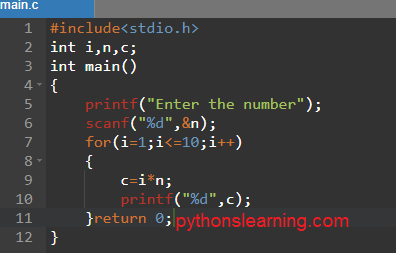For loop in c

## For loop in C vs Python | Basics of For loop

The looping simplifies complex programming logics to simple ones. Instead of writing the same code again and again with the help of for loop we can repeat the same code for a finite numbers of times.

1. Code re-usability possible
2. We do not need to write the same code again and again
3. We can Traverse over the elements of data structure

#### There are following loop supported by python

1. for loop
2. while loop
3. do_while loop

### For loop in python

• For loop is used in case where we need to execute some part of the code until the given condition is satisfied
• It is also called pre-tested loops
• It is better to use for loop if the number of iteration is known in advanced.

#### Syntax and python for loop codes

``````for interating_var in sequence:
statements(s)``````
``````list=[1,2,3,4,5,6,7,8,9,10]
n=5
for i in list:
c=n*i
print(c)``````
``[5,10,15,20,25,30,35,40,45,50]``

### For loop in c

Syntax and c program for loop

``````#include<stdio.h>
int i,n,c;
int main()
{
printf("Enter the number");
scanf("%d",&n);
for(i=1;i<=10;i++)
{
c=i*n;
printf("%d",c);
return 0;
}
}``````

Summary: